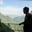Related Tags

r
communitycreator

# What are data frames in R?AKASH BAJWA

A data frame is an important data type in R. Data frames are the de facto data structure for tabular data and are used for statistics.

A data frame is a special type of list in which every element has an equal length. In other words, a data frame is a rectangular list.

Data frames have additional attributes, such as rownames(), that are useful for annotating data as subject_id or sample_id.

### Characteristics of data frames

• The column names should not be empty.
• The row name should be unique.
• The data stored in a data frame should be numeric, factor(dataType in R), or character type.
• Each column should contain the same number of data items.

### Explanation

You can create data frames with the read.csv() or read.table() method.

For example, importing the data into R, let’s assume all the columns in a data frame are of the same type. You can convert the data frame to a matrix with data.matix() or as.matrix().

We can also create a new data frame with the built-in data.frame() function. In addition, we can find the number of rows and columns by using and passing the data frame as an argument, i.e., nrow(frame), ncol(frame).

### Built-in methods

Here are some useful built-in methods that help to easily process data frames:

• nrow(): Denotes the number of rows
• ncol(): Denotes the number of columns
• head(): Denotes the first 6 rows
• tail(): Denotes the last 6 rows
• dim(): Gives the dimensions of the data frame, such as the number of rows and columns
• names() or colnames(): Shows the names of the attributes for a data frame
• str(): Defines the structure of the data frame as name, type, etc.
• sapply(dataframe, class): Denotes the class of each column in the data frame

The table below summarizes the one-dimensional and two-dimensional data structures in R by showing the relation of the diversity of data types:

Dimensions Homogenous Heterogenous
1-D Atomic Vector List
2-D Matix Data frame

### Example

In the example below, we initialize a data frame with 3 columns and 5 rows. The first column represents Index, while the other two are keys and their parallel values.

# Generating Data Frame in R
data_frame<-data.frame(Index=LETTERS[1:5],key=1:5,value=6:10)
cat('DEMO Dataframe \n')
print(data_frame)

# demo code for basic methods
cat('No. Of Rows \n')
nrow(data_frame)
cat('No. Of Cols \n')
ncol(data_frame)
cat('First 6 values from data Frame \n')
cat('Last 6 values from data Frame \n')
tail(data_frame)
cat('Dimentions of data frame \n')
dim(data_frame)
cat('Column names of data frame \n')
names(data_frame)
cat('structure of data frame \n')
str(data_frame)
cat('Show each Column dataType \n')
sapply(data_frame, class)

RELATED TAGS

r
communitycreator

CONTRIBUTORAKASH BAJWA
RELATED COURSES

View all Courses

Keep Exploring

Learn in-demand tech skills in half the time